Hypothesis Testing with Two Samples

# 49 Comparing Two Independent Population Means

The comparison of two independent population means is very common and provides a way to test the hypothesis that the two groups differ from each other. Is the night shift less productive than the day shift, are the rates of return from fixed asset investments different from those from common stock investments, and so on? An observed difference between two sample means depends on both the means and the sample standard deviations. Very different means can occur by chance if there is great variation among the individual samples. The test statistic will have to account for this fact. The test comparing two independent population means with unknown and possibly unequal population standard deviations is called the Aspin-Welch t-test. The degrees of freedom formula we will see later was developed by Aspin-Welch.

When we developed the hypothesis test for the mean and proportions we began with the Central Limit Theorem. We recognized that a sample mean came from a distribution of sample means, and sample proportions came from the sampling distribution of sample proportions. This made our sample parameters, the sample means and sample proportions, into random variables. It was important for us to know the distribution that these random variables came from. The Central Limit Theorem gave us the answer: the normal distribution. Our Z and t statistics came from this theorem. This provided us with the solution to our question of how to measure the probability that a sample mean came from a distribution with a particular hypothesized value of the mean or proportion. In both cases that was the question: what is the probability that the mean (or proportion) from our sample data came from a population distribution with the hypothesized value we are interested in?

Now we are interested in whether or not two samples have the same mean. Our question has not changed: Do these two samples come from the same population distribution? To approach this problem we create a new random variable. We recognize that we have two sample means, one from each set of data, and thus we have two random variables coming from two unknown distributions. To solve the problem we create a new random variable, the difference between the sample means. This new random variable also has a distribution and, again, the Central Limit Theorem tells us that this new distribution is normally distributed, regardless of the underlying distributions of the original data. A graph may help to understand this concept.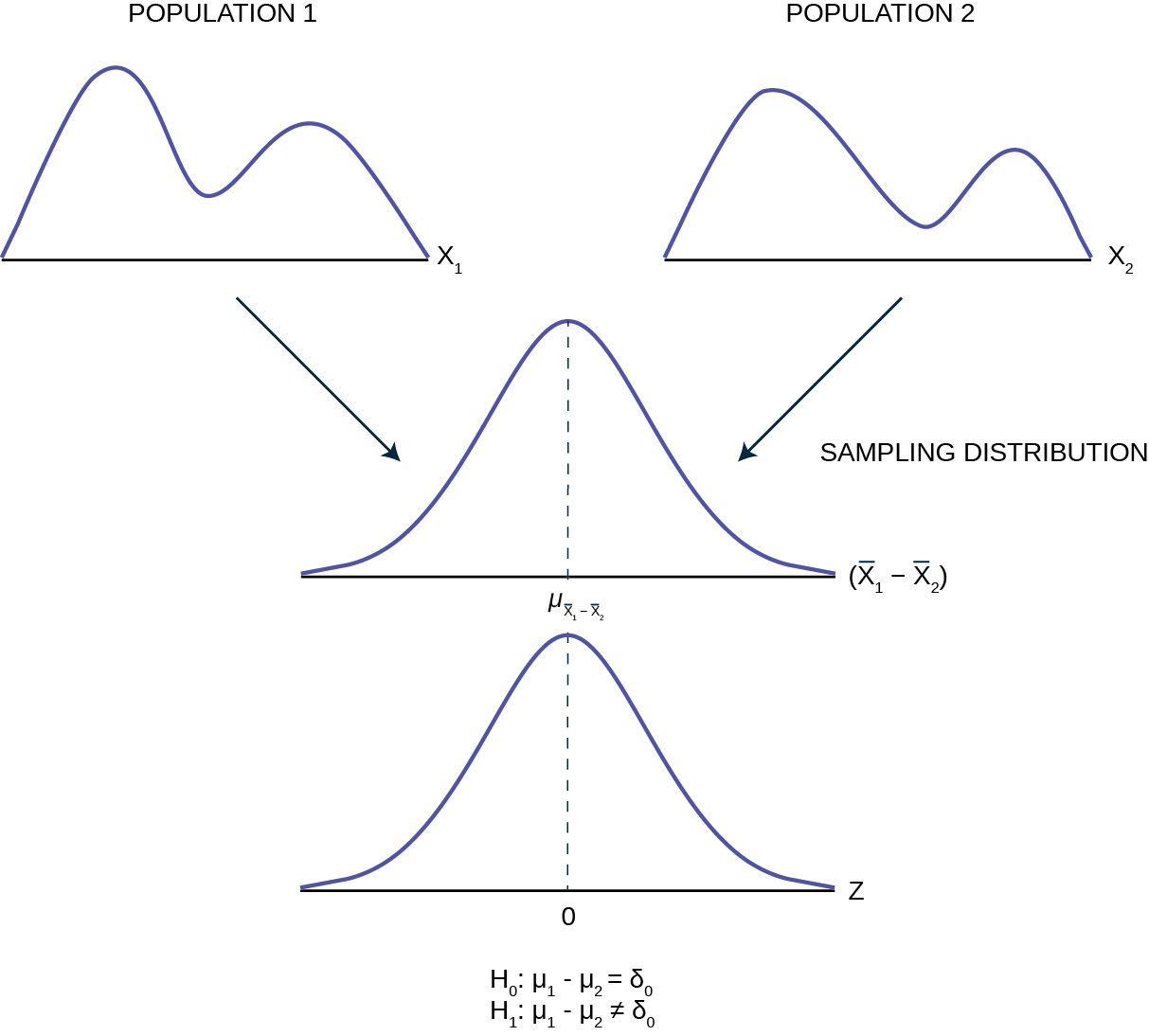Pictured are two distributions of data, X1 and X2, with unknown means and standard deviations. The second panel shows the sampling distribution of the newly created random variable (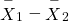). This distribution is the theoretical distribution of many many sample means from population 1 minus sample means from population 2. The Central Limit Theorem tells us that this theoretical sampling distribution of differences in sample means is normally distributed, regardless of the distribution of the actual population data shown in the top panel. Because the sampling distribution is normally distributed, we can develop a standardizing formula and calculate probabilities from the standard normal distribution in the bottom panel, the Z distribution. We have seen this same analysis before in Chapter 7 Figure 7.2 .

The Central Limit Theorem, as before, provides us with the standard deviation of the sampling distribution, and further, that the expected value of the mean of the distribution of differences in sample means is equal to the differences in the population means. Mathematically this can be stated: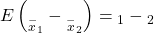Because we do not know the population standard deviations, we estimate them using the two sample standard deviations from our independent samples. For the hypothesis test, we calculate the estimated standard deviation, or standard error, of the difference in sample means,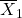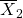.

The standard error is: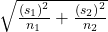We remember that substituting the sample variance for the population variance when we did not have the population variance was the technique we used when building the confidence interval and the test statistic for the test of hypothesis for a single mean back in Confidence Intervals and Hypothesis Testing with One Sample. The test statistic (t-score) is calculated as follows: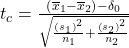where:
• s1 and s2, the sample standard deviations, are estimates of σ1 and σ2, respectively and
• σ1 and σ1 are the unknown population standard deviations.
•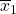and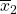are the sample means. μ1 and μ2 are the unknown population means.

The number of degrees of freedom (df) requires a somewhat complicated calculation. The df are not always a whole number. The test statistic above is approximated by the Student’s t-distribution with df as follows:

Degrees of freedom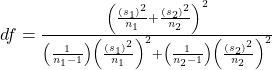When both sample sizes n1 and n2 are 30 or larger, the Student’s t approximation is very good. If each sample has more than 30 observations then the degrees of freedom can be calculated as n1 + n2 – 2.

The format of the sampling distribution, differences in sample means, specifies that the format of the null and alternative hypothesis is: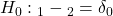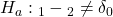where δ0 is the hypothesized difference between the two means. If the question is simply “is there any difference between the means?” then δ0 = 0 and the null and alternative hypotheses becomes: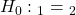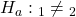An example of when δ0 might not be zero is when the comparison of the two groups requires a specific difference for the decision to be meaningful. Imagine that you are making a capital investment. You are considering changing from your current model machine to another. You measure the productivity of your machines by the speed they produce the product. It may be that a contender to replace the old model is faster in terms of product throughput, but is also more expensive. The second machine may also have more maintenance costs, setup costs, etc. The null hypothesis would be set up so that the new machine would have to be better than the old one by enough to cover these extra costs in terms of speed and cost of production. This form of the null and alternative hypothesis shows how valuable this particular hypothesis test can be. For most of our work we will be testing simple hypotheses asking if there is any difference between the two distribution means.

Independent groups

The Kona Iki Corporation produces coconut milk. They take coconuts and extract the milk inside by drilling a hole and pouring the milk into a vat for processing. They have both a day shift (called the B shift) and a night shift (called the G shift) to do this part of the process. They would like to know if the day shift and the night shift are equally efficient in processing the coconuts. A study is done sampling 9 shifts of the G shift and 16 shifts of the B shift. The results of the number of hours required to process 100 pounds of coconuts is presented in (Figure). A study is done and data are collected, resulting in the data in (Figure).

Sample Size Average Number of Hours to Process 100 Pounds of Coconuts Sample Standard Deviation
G Shift 9 2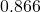B Shift 16 3.2 1.00

Is there a difference in the mean amount of time for each shift to process 100 pounds of coconuts? Test at the 5% level of significance.

The population standard deviations are not known and cannot be assumed to equal each other. Let g be the subscript for the G Shift and b be the subscript for the B Shift. Then, μg is the population mean for G Shift and μb is the population mean for B Shift. This is a test of two independent groups, two population means.

Random variable: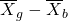= difference in the sample mean amount of time between the G Shift and the B Shift takes to process the coconuts.
H0: μg = μb  H0: μgμb = 0
Ha: μgμb  Ha: μgμb ≠ 0
The words “the same” tell you H0 has an “=”. Since there are no other words to indicate Ha, is either faster or slower. This is a two tailed test.

Distribution for the test: Use tdf where df is calculated using the df formula for independent groups, two population means above. Using a calculator, df is approximately 18.8462.

Graph: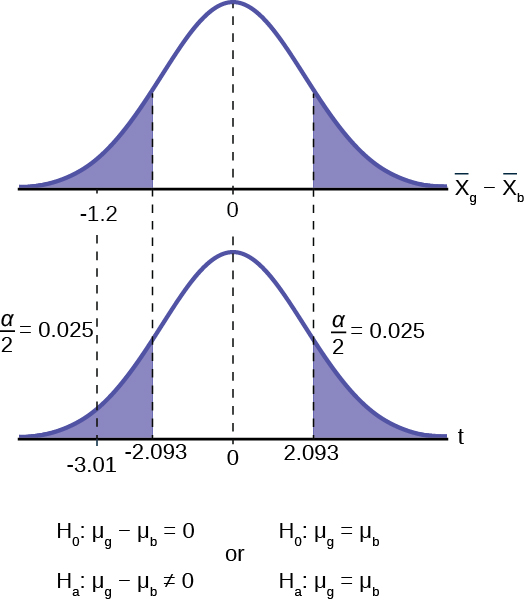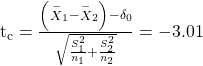We next find the critical value on the t-table using the degrees of freedom from above. The critical value, 2.093, is found in the .025 column, this is α/2, at 19 degrees of freedom. (The convention is to round up the degrees of freedom to make the conclusion more conservative.) Next we calculate the test statistic and mark this on the t-distribution graph.

Make a decision: Since the calculated t-value is in the tail we cannot accept the null hypothesis that there is no difference between the two groups. The means are different.

The graph has included the sampling distribution of the differences in the sample means to show how the t-distribution aligns with the sampling distribution data. We see in the top panel that the calculated difference in the two means is -1.2 and the bottom panel shows that this is 3.01 standard deviations from the mean. Typically we do not need to show the sampling distribution graph and can rely on the graph of the test statistic, the t-distribution in this case, to reach our conclusion.

Conclusion: At the 5% level of significance, the sample data show there is sufficient evidence to conclude that the mean number of hours that the G Shift takes to process 100 pounds of coconuts is different from the B Shift (mean number of hours for the B Shift is greater than the mean number of hours for the G Shift).

NOTE

When the sum of the sample sizes is larger than 30 (n1 + n2 > 30) you can use the normal distribution to approximate the Student’s t.

A study is done to determine if Company A retains its workers longer than Company B. It is believed that Company A has a higher retention than Company B. The study finds that in a sample of 11 workers at Company A their average time with the company is four years with a standard deviation of 1.5 years. A sample of 9 workers at Company B finds that the average time with the company was 3.5 years with a standard deviation of 1 year. Test this proposition at the 1% level of significance.

a. Is this a test of two means or two proportions?

a. two means because time is a continuous random variable.

b. Are the populations standard deviations known or unknown?

b. unknown

c. Which distribution do you use to perform the test?

c. Student’s t

d. What is the random variable?

d.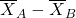e. What are the null and alternate hypotheses?

e.

•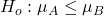•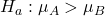f. Is this test right-, left-, or two-tailed?

f. right one-tailed test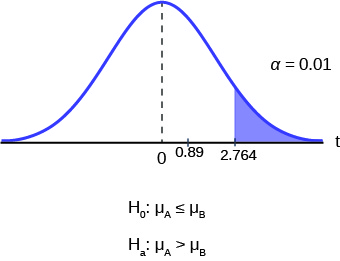g. What is the value of the test statistic?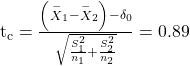h. Can you accept/reject the null hypothesis?

h. Cannot reject the null hypothesis that there is no difference between the two groups. Test statistic is not in the tail. The critical value of the t distribution is 2.764 with 10 degrees of freedom. This example shows how difficult it is to reject a null hypothesis with a very small sample. The critical values require very large test statistics to reach the tail.

i. Conclusion:

i. At the 1% level of significance, from the sample data, there is not sufficient evidence to conclude that the retention of workers at Company A is longer than Company B, on average.

An interesting research question is the effect, if any, that different types of teaching formats have on the grade outcomes of students. To investigate this issue one sample of students’ grades was taken from a hybrid class and another sample taken from a standard lecture format class. Both classes were for the same subject. The mean course grade in percent for the 35 hybrid students is 74 with a standard deviation of 16. The mean grades of the 40 students form the standard lecture class was 76 percent with a standard deviation of 9. Test at 5% to see if there is any significant difference in the population mean grades between standard lecture course and hybrid class.

We begin by noting that we have two groups, students from a hybrid class and students from a standard lecture format class. We also note that the random variable, what we are interested in, is students’ grades, a continuous random variable. We could have asked the research question in a different way and had a binary random variable. For example, we could have studied the percentage of students with a failing grade, or with an A grade. Both of these would be binary and thus a test of proportions and not a test of means as is the case here. Finally, there is no presumption as to which format might lead to higher grades so the hypothesis is stated as a two-tailed test.

H0: µ1 = µ2
Ha: µ1µ2

As would virtually always be the case, we do not know the population variances of the two distributions and thus our test statistic is: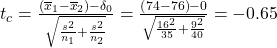To determine the critical value of the Student’s t we need the degrees of freedom. For this case we use: df = n1 + n2 – 2 = 35 + 40 -2 = 73. This is large enough to consider it the normal distribution thus ta/2 = 1.96. Again as always we determine if the calculated value is in the tail determined by the critical value. In this case we do not even need to look up the critical value: the calculated value of the difference in these two average grades is not even one standard deviation apart. Certainly not in the tail.

Conclusion: Cannot reject the null at α=5%. Therefore, evidence does not exist to prove that the grades in hybrid and standard classes differ.

### References

Data from Microsoft Bookshelf.

Data from the United States Senate website, available online at www.Senate.gov (accessed June 17, 2013).

“List of current United States Senators by Age.” Wikipedia. Available online at http://en.wikipedia.org/wiki/List_of_current_United_States_Senators_by_age (accessed June 17, 2013).

“Sectoring by Industry Groups.” Nasdaq. Available online at http://www.nasdaq.com/markets/barchart-sectors.aspx?page=sectors&base=industry (accessed June 17, 2013).

“Strip Clubs: Where Prostitution and Trafficking Happen.” Prostitution Research and Education, 2013. Available online at www.prostitutionresearch.com/ProsViolPosttrauStress.html (accessed June 17, 2013).

“World Series History.” Baseball-Almanac, 2013. Available online at http://www.baseball-almanac.com/ws/wsmenu.shtml (accessed June 17, 2013).

### Chapter Review

Two population means from independent samples where the population standard deviations are not known

• Random Variable:= the difference of the sampling means
• Distribution: Student’s t-distribution with degrees of freedom (variances not pooled)

### Formula Review

Standard error: SE =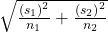Test statistic (t-score): tc =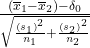Degrees of freedom: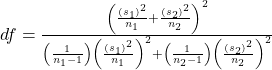where: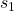and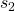are the sample standard deviations, and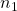and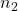are the sample sizes.andare the sample means.

Use the following information to answer the next 15 exercises: Indicate if the hypothesis test is for

1. independent group means, population standard deviations, and/or variances known
2. independent group means, population standard deviations, and/or variances unknown
3. matched or paired samples
4. single mean
5. two proportions
6. single proportion

It is believed that 70% of males pass their drivers test in the first attempt, while 65% of females pass the test in the first attempt. Of interest is whether the proportions are in fact equal.

two proportions

A new laundry detergent is tested on consumers. Of interest is the proportion of consumers who prefer the new brand over the leading competitor. A study is done to test this.

A new windshield treatment claims to repel water more effectively. Ten windshields are tested by simulating rain without the new treatment. The same windshields are then treated, and the experiment is run again. A hypothesis test is conducted.

matched or paired samples

The known standard deviation in salary for all mid-level professionals in the financial industry is ?11,000. Company A and Company B are in the financial industry. Suppose samples are taken of mid-level professionals from Company A and from Company B. The sample mean salary for mid-level professionals in Company A is ?80,000. The sample mean salary for mid-level professionals in Company B is ?96,000. Company A and Company B management want to know if their mid-level professionals are paid differently, on average.

The average worker in Germany gets eight weeks of paid vacation.

single mean

According to a television commercial, 80% of dentists agree that Ultrafresh toothpaste is the best on the market.

It is believed that the average grade on an English essay in a particular school system for females is higher than for males. A random sample of 31 females had a mean score of 82 with a standard deviation of three, and a random sample of 25 males had a mean score of 76 with a standard deviation of four.

independent group means, population standard deviations and/or variances unknown

The league mean batting average is 0.280 with a known standard deviation of 0.06. The Rattlers and the Vikings belong to the league. The mean batting average for a sample of eight Rattlers is 0.210, and the mean batting average for a sample of eight Vikings is 0.260. There are 24 players on the Rattlers and 19 players on the Vikings. Are the batting averages of the Rattlers and Vikings statistically different?

In a random sample of 100 forests in the United States, 56 were coniferous or contained conifers. In a random sample of 80 forests in Mexico, 40 were coniferous or contained conifers. Is the proportion of conifers in the United States statistically more than the proportion of conifers in Mexico?

two proportions

A new medicine is said to help improve sleep. Eight subjects are picked at random and given the medicine. The means hours slept for each person were recorded before starting the medication and after.

It is thought that teenagers sleep more than adults on average. A study is done to verify this. A sample of 16 teenagers has a mean of 8.9 hours slept and a standard deviation of 1.2. A sample of 12 adults has a mean of 6.9 hours slept and a standard deviation of 0.6.

independent group means, population standard deviations and/or variances unknown

Varsity athletes practice five times a week, on average.

A sample of 12 in-state graduate school programs at school A has a mean tuition of ?64,000 with a standard deviation of ?8,000. At school B, a sample of 16 in-state graduate programs has a mean of ?80,000 with a standard deviation of ?6,000. On average, are the mean tuitions different?

independent group means, population standard deviations and/or variances unknown

A new WiFi range booster is being offered to consumers. A researcher tests the native range of 12 different routers under the same conditions. The ranges are recorded. Then the researcher uses the new WiFi range booster and records the new ranges. Does the new WiFi range booster do a better job?

A high school principal claims that 30% of student athletes drive themselves to school, while 4% of non-athletes drive themselves to school. In a sample of 20 student athletes, 45% drive themselves to school. In a sample of 35 non-athlete students, 6% drive themselves to school. Is the percent of student athletes who drive themselves to school more than the percent of nonathletes?

two proportions

Use the following information to answer the next three exercises: A study is done to determine which of two soft drinks has more sugar. There are 13 cans of Beverage A in a sample and six cans of Beverage B. The mean amount of sugar in Beverage A is 36 grams with a standard deviation of 0.6 grams. The mean amount of sugar in Beverage B is 38 grams with a standard deviation of 0.8 grams. The researchers believe that Beverage B has more sugar than Beverage A, on average. Both populations have normal distributions.

Are standard deviations known or unknown?

What is the random variable?

The random variable is the difference between the mean amounts of sugar in the two soft drinks.

Is this a one-tailed or two-tailed test?

Use the following information to answer the next 12 exercises: The U.S. Center for Disease Control reports that the mean life expectancy was 47.6 years for whites born in 1900 and 33.0 years for nonwhites. Suppose that you randomly survey death records for people born in 1900 in a certain county. Of the 124 whites, the mean life span was 45.3 years with a standard deviation of 12.7 years. Of the 82 nonwhites, the mean life span was 34.1 years with a standard deviation of 15.6 years. Conduct a hypothesis test to see if the mean life spans in the county were the same for whites and nonwhites.

Is this a test of means or proportions?

means

State the null and alternative hypotheses.

1. H0: __________
2. Ha: __________

Is this a right-tailed, left-tailed, or two-tailed test?

two-tailed

In symbols, what is the random variable of interest for this test?

In words, define the random variable of interest for this test.

the difference between the mean life spans of whites and nonwhites

Which distribution (normal or Student’s t) would you use for this hypothesis test?

Explain why you chose the distribution you did for (Figure).

This is a comparison of two population means with unknown population standard deviations.

Calculate the test statistic.

Sketch a graph of the situation. Label the horizontal axis. Mark the hypothesized difference and the sample difference. Shade the area corresponding to the p-value.

Check student’s solution.

At a pre-conceived α = 0.05, what is your:

1. Decision:
2. Reason for the decision:
3. Conclusion (write out in a complete sentence):
1. Cannot accept the null hypothesis
2. p-value < 0.05
3. There is not enough evidence at the 5% level of significance to support the claim that life expectancy in the 1900s is different between whites and nonwhites.

Does it appear that the means are the same? Why or why not?

### Homework

The mean number of English courses taken in a two–year time period by male and female college students is believed to be about the same. An experiment is conducted and data are collected from 29 males and 16 females. The males took an average of three English courses with a standard deviation of 0.8. The females took an average of four English courses with a standard deviation of 1.0. Are the means statistically the same?

A student at a four-year college claims that mean enrollment at four–year colleges is higher than at two–year colleges in the United States. Two surveys are conducted. Of the 35 two–year colleges surveyed, the mean enrollment was 5,068 with a standard deviation of 4,777. Of the 35 four-year colleges surveyed, the mean enrollment was 5,466 with a standard deviation of 8,191.

Subscripts: 1: two-year colleges; 2: four-year colleges

1.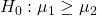2.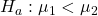3.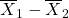is the difference between the mean enrollments of the two-year colleges and the four-year colleges.
4. Student’s-t
5. test statistic: -0.2480
6. p-value: 0.4019
7. Check student’s solution.
1. Alpha: 0.05
2. Decision: Cannot reject
3. Reason for Decision: p-value > alpha
4. Conclusion: At the 5% significance level, there is sufficient evidence to conclude that the mean enrollment at four-year colleges is higher than at two-year colleges.

At Rachel’s 11th birthday party, eight girls were timed to see how long (in seconds) they could hold their breath in a relaxed position. After a two-minute rest, they timed themselves while jumping. The girls thought that the mean difference between their jumping and relaxed times would be zero. Test their hypothesis.

Relaxed time (seconds) Jumping time (seconds)
26 21
47 40
30 28
22 21
23 25
45 43
37 35
29 32

Mean entry-level salaries for college graduates with mechanical engineering degrees and electrical engineering degrees are believed to be approximately the same. A recruiting office thinks that the mean mechanical engineering salary is actually lower than the mean electrical engineering salary. The recruiting office randomly surveys 50 entry level mechanical engineers and 60 entry level electrical engineers. Their mean salaries were ?46,100 and ?46,700, respectively. Their standard deviations were ?3,450 and ?4,210, respectively. Conduct a hypothesis test to determine if you agree that the mean entry-level mechanical engineering salary is lower than the mean entry-level electrical engineering salary.

Subscripts: 1: mechanical engineering; 2: electrical engineering

1.2.3.is the difference between the mean entry level salaries of mechanical engineers and electrical engineers.
4. t108
5. test statistic: t = –0.82
6. p-value: 0.2061
7. Check student’s solution.
1. Alpha: 0.05
2. Decision: Cannot reject the null hypothesis.
3. Reason for Decision: p-value > alpha
4. Conclusion: At the 5% significance level, there is insufficient evidence to conclude that the mean entry-level salaries of mechanical engineers is lower than that of electrical engineers.

Marketing companies have collected data implying that teenage girls use more ring tones on their cellular phones than teenage boys do. In one particular study of 40 randomly chosen teenage girls and boys (20 of each) with cellular phones, the mean number of ring tones for the girls was 3.2 with a standard deviation of 1.5. The mean for the boys was 1.7 with a standard deviation of 0.8. Conduct a hypothesis test to determine if the means are approximately the same or if the girls’ mean is higher than the boys’ mean.

Use the information from Appendix C: Data Sets to answer the next four exercises.

Using the data from Lap 1 only, conduct a hypothesis test to determine if the mean time for completing a lap in races is the same as it is in practices.

1.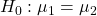2.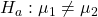3.is the difference between the mean times for completing a lap in races and in practices.
4. t20.32
5. test statistic: –4.70
6. p-value: 0.0001
7. Check student’s solution.
1. Alpha: 0.05
2. Decision: Cannot accept the null hypothesis.
3. Reason for Decision: p-value < alpha
4. Conclusion: At the 5% significance level, there is sufficient evidence to conclude that the mean time for completing a lap in races is different from that in practices.

Repeat the test in (Figure), but use Lap 5 data this time.

Repeat the test in (Figure), but this time combine the data from Laps 1 and 5.

1.2.3. is the difference between the mean times for completing a lap in races and in practices.
4. t40.94
5. test statistic: –5.08
6. p-value: zero
7. Check student’s solution.
1. Alpha: 0.05
2. Decision: Cannot accept the null hypothesis.
3. Reason for Decision: p-value < alpha
4. Conclusion: At the 5% significance level, there is sufficient evidence to conclude that the mean time for completing a lap in races is different from that in practices.

In two to three complete sentences, explain in detail how you might use Terri Vogel’s data to answer the following question. “Does Terri Vogel drive faster in races than she does in practices?”

Use the following information to answer the next two exercises. The Eastern and Western Major League Soccer conferences have a new Reserve Division that allows new players to develop their skills. Data for a randomly picked date showed the following annual goals.

Western Eastern
Los Angeles 9 D.C. United 9
FC Dallas 3 Chicago 8
Chivas USA 4 Columbus 7
Real Salt Lake 3 New England 6
San Jose 4 Kansas City 3

Conduct a hypothesis test to answer the next two exercises.

The exact distribution for the hypothesis test is:

1. the normal distribution
2. the Student’s t-distribution
3. the uniform distribution
4. the exponential distribution

If the level of significance is 0.05, the conclusion is:

1. There is sufficient evidence to conclude that the W Division teams score fewer goals, on average, than the E teams
2. There is insufficient evidence to conclude that the W Division teams score more goals, on average, than the E teams.
3. There is insufficient evidence to conclude that the W teams score fewer goals, on average, than the E teams score.
4. Unable to determine

c

Suppose a statistics instructor believes that there is no significant difference between the mean class scores of statistics day students on Exam 2 and statistics night students on Exam 2. She takes random samples from each of the populations. The mean and standard deviation for 35 statistics day students were 75.86 and 16.91. The mean and standard deviation for 37 statistics night students were 75.41 and 19.73. The “day” subscript refers to the statistics day students. The “night” subscript refers to the statistics night students. A concluding statement is:

1. There is sufficient evidence to conclude that statistics night students’ mean on Exam 2 is better than the statistics day students’ mean on Exam 2.
2. There is insufficient evidence to conclude that the statistics day students’ mean on Exam 2 is better than the statistics night students’ mean on Exam 2.
3. There is insufficient evidence to conclude that there is a significant difference between the means of the statistics day students and night students on Exam 2.
4. There is sufficient evidence to conclude that there is a significant difference between the means of the statistics day students and night students on Exam 2.

Researchers interviewed street prostitutes in Canada and the United States. The mean age of the 100 Canadian prostitutes upon entering prostitution was 18 with a standard deviation of six. The mean age of the 130 United States prostitutes upon entering prostitution was 20 with a standard deviation of eight. Is the mean age of entering prostitution in Canada lower than the mean age in the United States? Test at a 1% significance level.

Test: two independent sample means, population standard deviations unknown.

Random variable:Distribution: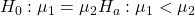H0: μ1 = μ2Ha: μ1 < μ2 The mean age of entering prostitution in Canada is lower than the mean age in the United States.

Graph: left-tailed

p-value : 0.0151

Decision: Cannot reject H0.

Conclusion: At the 1% level of significance, from the sample data, there is not sufficient evidence to conclude that the mean age of entering prostitution in Canada is lower than the mean age in the United States.

A powder diet is tested on 49 people, and a liquid diet is tested on 36 different people. Of interest is whether the liquid diet yields a higher mean weight loss than the powder diet. The powder diet group had a mean weight loss of 42 pounds with a standard deviation of 12 pounds. The liquid diet group had a mean weight loss of 45 pounds with a standard deviation of 14 pounds.

Suppose a statistics instructor believes that there is no significant difference between the mean class scores of statistics day students on Exam 2 and statistics night students on Exam 2. She takes random samples from each of the populations. The mean and standard deviation for 35 statistics day students were 75.86 and 16.91, respectively. The mean and standard deviation for 37 statistics night students were 75.41 and 19.73. The “day” subscript refers to the statistics day students. The “night” subscript refers to the statistics night students. An appropriate alternative hypothesis for the hypothesis test is:

1. μday > μnight
2. μday < μnight
3. μday = μnight
4. μdayμnight

d

### Key Terms

Cohen’s d
a measure of effect size based on the differences between two means. If d is between 0 and 0.2 then the effect is small. If d approaches is 0.5, then the effect is medium, and if d approaches 0.8, then it is a large effect.
Pooled Variance
a weighted average of two variances that can then be used when calculating standard error.